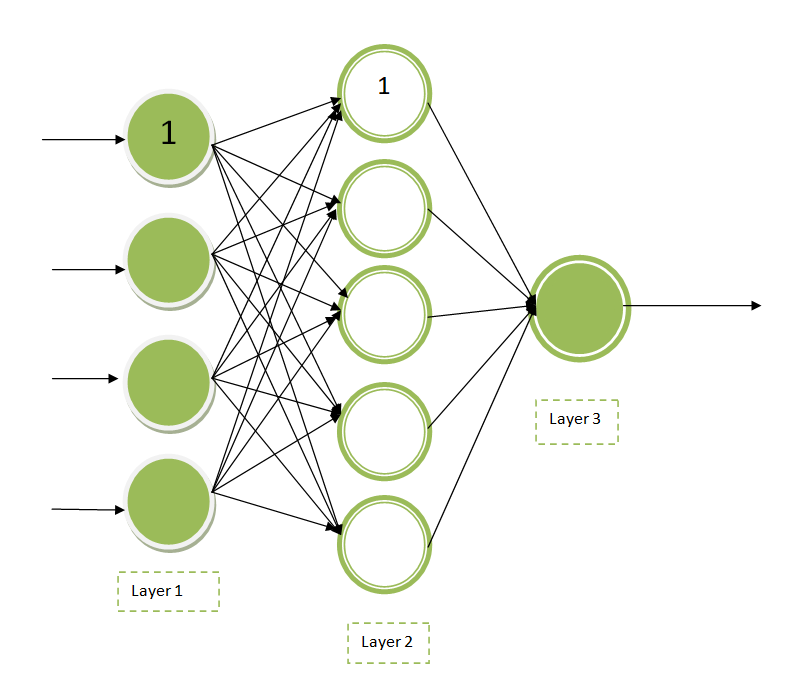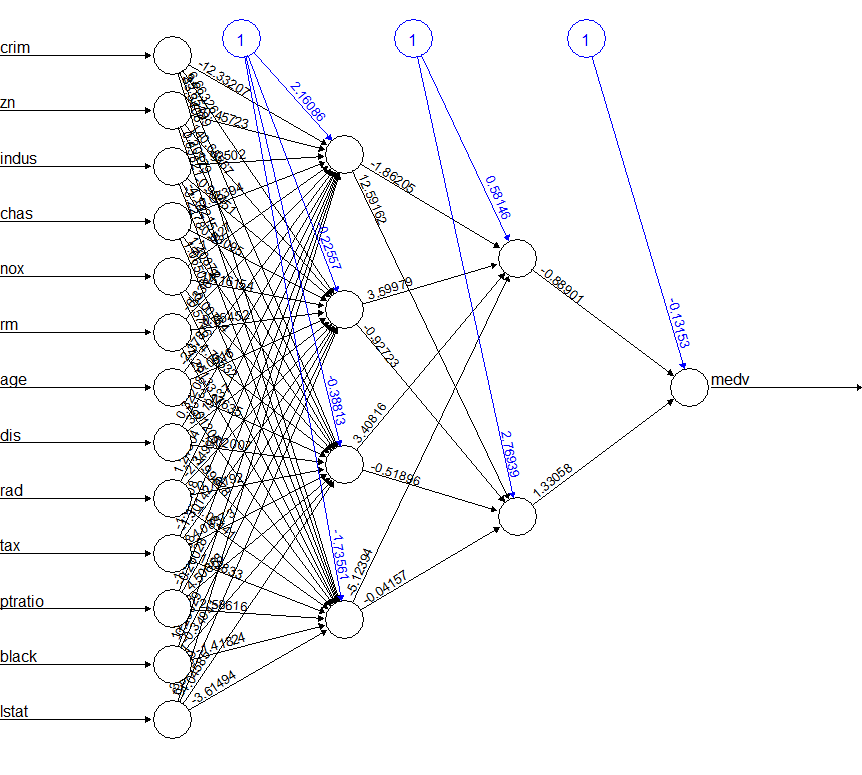# Multi Layered Neural Networks in R Programming

• Last Updated : 30 Jun, 2022

A series or set of algorithms that try to recognize the underlying relationship in a data set through a definite process that mimics the operation of the human brain is known as a Neural Network. Hence, the neural networks could refer to the neurons of the human, either artificial or organic in nature. A neural network can easily adapt to the changing input to achieve or generate the best possible result for the network and does not need to redesign the output criteria.

### Types of Neural Network

Neural Networks can be classified into multiple types based on their Layers and depth activation filters, Structure, Neurons used, Neuron density, data flow, and so on. The types of Neural Networks are as follows:

• Perceptron
• Multi-Layer Perceptron or Multi-Layer Neural Network
• Feed Forward Neural Networks
• Convolutional Neural Networks
• Radial Basis Function Neural Networks
• Recurrent Neural Networks
• Sequence to Sequence Model
• Modular Neural Network

#### Multi-Layer Neural Network

To be accurate a fully connected Multi-Layered Neural Network is known as Multi-Layer Perceptron. A Multi-Layered Neural Network consists of multiple layers of artificial neurons or nodes. Unlike Single-Layer Neural networks, in recent times most networks have Multi-Layered Neural Network. The following diagram is a visualization of a multi-layer neural network.Explanation: Here the nodes marked as “1” are known as bias units. The leftmost layer or Layer 1 is the input layer, the middle layer or Layer 2 is the hidden layer and the rightmost layer or Layer 3 is the output layer. It can say that the above diagram has 3 input units (leaving the bias unit), 1 output unit, and 4 hidden units(1 bias unit is not included).

A Multi-layered Neural Network is a typical example of the Feed Forward Neural Network. The number of neurons and the number of layers consists of the hyperparameters of Neural Networks which need tuning. In order to find ideal values for the hyperparameters, one must use some cross-validation techniques. Using the Back-Propagation technique, weight adjustment training is carried out.

### Formula for Multi-Layered Neural Network

Suppose we have xn inputs(x1, x2….xn) and a bias unit. Let the weight applied to be w1, w2…..wn. Then find the summation and bias unit on performing dot product among inputs and weights as:

`r = Σmi=1 wixi + bias`

On feeding the r into activation function F(r) we find the output for the hidden layers. For the first hidden layer h1, the neuron can be calculated as:

`h11 = F(r)`

For all the other hidden layers repeat the same procedure. Keep repeating the process until reach the last weight set.

### Implementing Multi-Layered Neural Network in R

In R Language, install the neuralnet package to work on the concepts of Neural Network. The neuralnet package demands an all-numeric matrix or data frame. Control the hidden layers by mentioning the value against the hidden parameter of the neuralnet() function which can be a vector for many hidden layers. Use the set.seed() function every time to generate random numbers.

Example: Use the neuralnet package in order to fit a linear model. Let us see the steps to fit a Multi-Layered Neural network in R.

Step 1: The first step is to pick the dataset. Here in this example, let’s work on the Boston dataset of the MASS package. This dataset typically deals with the housing values in the fringes or suburbs of Boston. The goal is to find the medv or median values of the houses occupied by its owner by using all the other available continuous variables. Use the set.seed() function to generate random numbers.

## r

 `set.seed``(500)` `library``(MASS)` `data <- Boston`

Step 2: Then check for missing values or data points in the dataset. If any, then fix the data points which are missing.

## r

 `apply``(data, 2, ``function``(x) ``sum``(``is.na``(x)))`

Output:

```crim      zn   indus    chas     nox      rm     age     dis     rad     tax ptratio   black   lstat    medv
0       0       0       0       0       0       0       0       0       0       0       0       0       0 ```

Step 3: Since no data points are missing proceed towards preparing the data set. Now randomly split the data into two sets, Train set and Test set. On preparing the data, try to fit the data on a linear regression model and then test it on the test set.

## r

 `index <- ``sample``(1 : ``nrow``(data), ` `                ``round``(0.75 * ``nrow``(data)))` `train <- data[index, ]` `test <- data[-index, ]` `lm.fit <- ``glm``(medv~., data = train)` `summary``(lm.fit)` `pr.lm <- ``predict``(lm.fit, test)` `MSE.lm <- ``sum``((pr.lm - test\$medv)^2) / ``nrow``(test)`

Output:

```Deviance Residuals:
Min        1Q    Median        3Q       Max
-14.9143   -2.8607   -0.5244    1.5242   25.0004

Coefficients:
Estimate Std. Error t value Pr(>|t|)
(Intercept)  43.469681   6.099347   7.127 5.50e-12 ***
crim         -0.105439   0.057095  -1.847 0.065596 .
zn            0.044347   0.015974   2.776 0.005782 **
indus         0.024034   0.071107   0.338 0.735556
chas          2.596028   1.089369   2.383 0.017679 *
nox         -22.336623   4.572254  -4.885 1.55e-06 ***
rm            3.538957   0.472374   7.492 5.15e-13 ***
age           0.016976   0.015088   1.125 0.261291
dis          -1.570970   0.235280  -6.677 9.07e-11 ***
rad           0.400502   0.085475   4.686 3.94e-06 ***
tax          -0.015165   0.004599  -3.297 0.001072 **
ptratio      -1.147046   0.155702  -7.367 1.17e-12 ***
black         0.010338   0.003077   3.360 0.000862 ***
lstat        -0.524957   0.056899  -9.226  < 2e-16 ***
---
Signif. codes:  0 ‘***’ 0.001 ‘**’ 0.01 ‘*’ 0.05 ‘.’ 0.1 ‘ ’ 1

(Dispersion parameter for gaussian family taken to be 23.26491)

Null deviance: 33642  on 379  degrees of freedom
Residual deviance:  8515  on 366  degrees of freedom
AIC: 2290

Number of Fisher Scoring iterations: 2```

Step 4: Now normalize the data set before training a Neural network. Hence, scaling and splitting the data. The scale() function returns a matrix that needs to be coerced in data frame.

## r

 `maxs <- ``apply``(data, 2, max) ` `mins <- ``apply``(data, 2, min)` `scaled <- ``as.data.frame``(``scale``(data, ` `                              ``center = mins,` `                              ``scale = maxs - mins))` `train_ <- scaled[index, ]` `test_ <- scaled[-index, ]`

Step 5: Now fit the data into Neural network. Use the neuralnet package.

## r

 `library``(neuralnet)` `n <- ``names``(train_)` `f <- ``as.formula``(``paste``(``"medv ~"``, ` `                ``paste``(n[!n %``in``% ``"medv"``],` `                ``collapse = ``" + "``)))` `nn <- ``neuralnet``(f, ` `               ``data = train_, ` `               ``hidden = ``c``(4, 2), ` `               ``linear.output = T)`

Now our model is fitted to the Multi-Layered Neural network. Now combine all the steps and also plot the neural network to visualize the output. Use the plot() function to do so.

## r

 `# R program to illustrate` `# Multi Layered Neural Networks`   `# Use the set.seed() function` `# To generate random numbers` `set.seed``(500)`   `# Import required library` `library``(MASS)`   `# Working on the Boston dataset` `data <- Boston` `apply``(data, 2, ``function``(x) ``sum``(``is.na``(x)))` `index <- ``sample``(1 : ``nrow``(data), ` `                ``round``(0.75 * ``nrow``(data)))` `train <- data[index, ]` `test <- data[-index, ]` `lm.fit <- ``glm``(medv~., data = train)` `summary``(lm.fit)` `pr.lm <- ``predict``(lm.fit, test)` `MSE.lm <- ``sum``((pr.lm - test\$medv)^2) / ``nrow``(test)` `maxs <- ``apply``(data, 2, max) ` `mins <- ``apply``(data, 2, min)` `scaled <- ``as.data.frame``(``scale``(data, ` `                              ``center = mins, ` `                              ``scale = maxs - mins))` `train_ <- scaled[index, ]` `test_ <- scaled[-index, ]`   `# Applying Neural network concepts` `library``(neuralnet)` `n <- ``names``(train_)` `f <- ``as.formula``(``paste``(``"medv ~"``, ` `                       ``paste``(n[!n %``in``% ``"medv"``],` `                       ``collapse = ``" + "``)))` `nn <- ``neuralnet``(f, data = train_, ` `                   ``hidden = ``c``(4, 2), ` `                   ``linear.output = T)`   `# Plotting the graph` `plot``(nn)`

Output:My Personal Notes arrow_drop_up
Recommended Articles
Page :